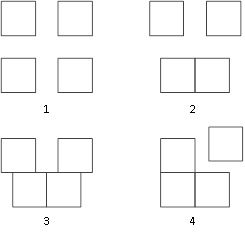### Sample Problem

Ivor has many ways to place 4 identical 3 cm × 3 cm squares together as follow:Among these figures, compare the perimeters of all the figures. Which figure’s perimeter is the longest? Answer 1, 2, 3, or 4.

#### Solution

The perimeter of (1) is the longest because none of the squares share sides with one another. The total perimeter of the figure is 3 × 4 × 4 = 48 cm.

In general, the less shared segments that a figure has, the longer its perimeter, as you will find out in the rest of this section.